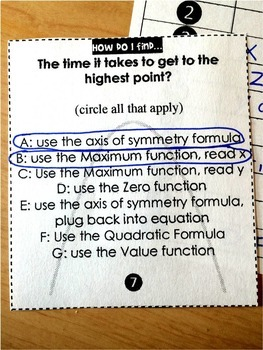digital

# Numberless Quadratic Word Problems Activity - print and digitalSubject
Resource Type
Format
PDF (1 MB|5 pages)Google Apps™
StandardsThe Teacher-Author indicated this resource includes assets from Google Workspace (eg. docs, slides, etc.).

#### Also included in

1. This bundle contains all of the resources you will need to teach a summer school algebra class. Resources inside cover: solving equations, slope & linear equations, functions & domain and range, polynomials, systems of equations, factoring quadratics and the quadratic formula. For a bundle o
\$34.00
\$51.00
Save \$17.00
2. This bundle includes a variety of activities, resources and supports for your quadratics unit. Factoring quadratics, using algebra tiles, solving quadratic word problems, the Quadratic Formula and graphing quadratic functions. You may also like:Algebra Activities Bundle w/ digital updates
\$36.50
\$53.50
Save \$17.00

### Description

*Includes both printable and digital GOOGLE Slides versions.

In this quadratics activity, students have to decide if a word problem is asking them to find the x at the vertex, the y at the vertex or the positive zero of a parabola. There are no numbers or problems to solve, which forces students to look for keywords to determine which part of the parabola a word problem is asking them to find. This activity helps students remember to look for the keywords in future quadratic word problems.

This activity works well during a projectile motion unit to help familiarize students with the wording used in word problems. Includes both print and digital.

You may also like:

Factoring Quadratics Digital Math Escape Room

Total Pages
5 pages
Included
Teaching Duration
N/A
Report this Resource to TpT
Reported resources will be reviewed by our team. Report this resource to let us know if this resource violates TpT’s content guidelines.

### Standards

to see state-specific standards (only available in the US).
Use the process of factoring and completing the square in a quadratic function to show zeros, extreme values, and symmetry of the graph, and interpret these in terms of a context.
Graph linear and quadratic functions and show intercepts, maxima, and minima.
Graph functions expressed symbolically and show key features of the graph, by hand in simple cases and using technology for more complicated cases.
Create equations in two or more variables to represent relationships between quantities; graph equations on coordinate axes with labels and scales.